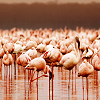# Number

This is part of our Secondary Curriculum collection of favourite rich tasks arranged by topic.### Place Value, Integers, Ordering & Rounding

Working on these problems will help your students develop a better understanding of place value, ordering and rounding.### Factors, Multiples and Primes

Working on these problems will help your students develop a better understanding of factors, multiples and primes.### Powers and Roots### Fractions, Decimals & Percentages

Working on these problems will help your students develop a better understanding of fractions, decimals and percentages.### Number Operations & Calculation Methods

Working on these problems will help your students develop a better understanding of number operations and calculation methods.### Ratio, Proportion & Rates of Change

Working on these problems will help your students develop a better understanding of ratio, proportion and rates of change.